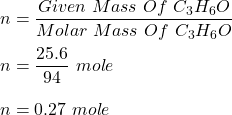## How many liters of C3H6O are present in a sample weighing 25.6 grams?

Question

How many liters of C3H6O are present in a sample weighing 25.6 grams?

in progress 0
6 months 2021-07-30T04:31:42+00:00 1 Answers 3 views 0

1. To Find :

Number of moles of C₃H₆O present in a sample weighing 25.6 grams.

Solution :

Molecular mass of C₃H₆O is :

M = (6×12) + (6×1) + (16×1) grams

M = 94 grams/mol

We know, number of moles of 25.6 grams of C₃H₆O is :Hence, this is the required solution.